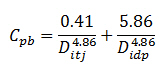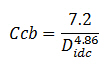# Coefficient For ID of Pipe and Tool Joints

You’ve learned about pressure loss in the drill string from the previous topic (Pressure Losses through Drill String Calculation). The general coefficient for internal diameter of pipe does not account the inside diameter of tool joint.

The general coefficient equation is shown below:Coefficient of The Bore of Drill Pipe and Tool Joint

To get the accurate pressure loss calculation, the coefficient of a section of pipe must be accounted for both the pipe ID and the tool joint ID.In general, each 100 ft of pipe, there is appoximately 6.7 ft of tool joint length (6.7% of total length) and 93.3 ft of drill pipe (93.3% of total length). Therefore, the general coefficient for internal diameter of pipe can be derived in to this following equation:Where;

Cpb = coefficient through inside of pipe and tool joint

Ditj = internal diameter of tool joint, inch

Didp = internal diameter of drill pipe, inch

Coefficient of The Drill Collar

The coefficient of drill collar increases by 18% to compensate for higher roughness in drill collars. The new equation for coefficient of drill collar is listed below:Where;

Ccb = coefficient through inside of drill collar

Didc = internal diameter of drill collar, inch

With new coefficients (coefficient of drill pipe and drill collar), the new equations for pressure drop through pipe are as follows:

Pressure drop through inside of drill pipePressure drop through inside of drill collarReference:Drilling Hydraulic Books

Share the joy1.hamza victor habib says: